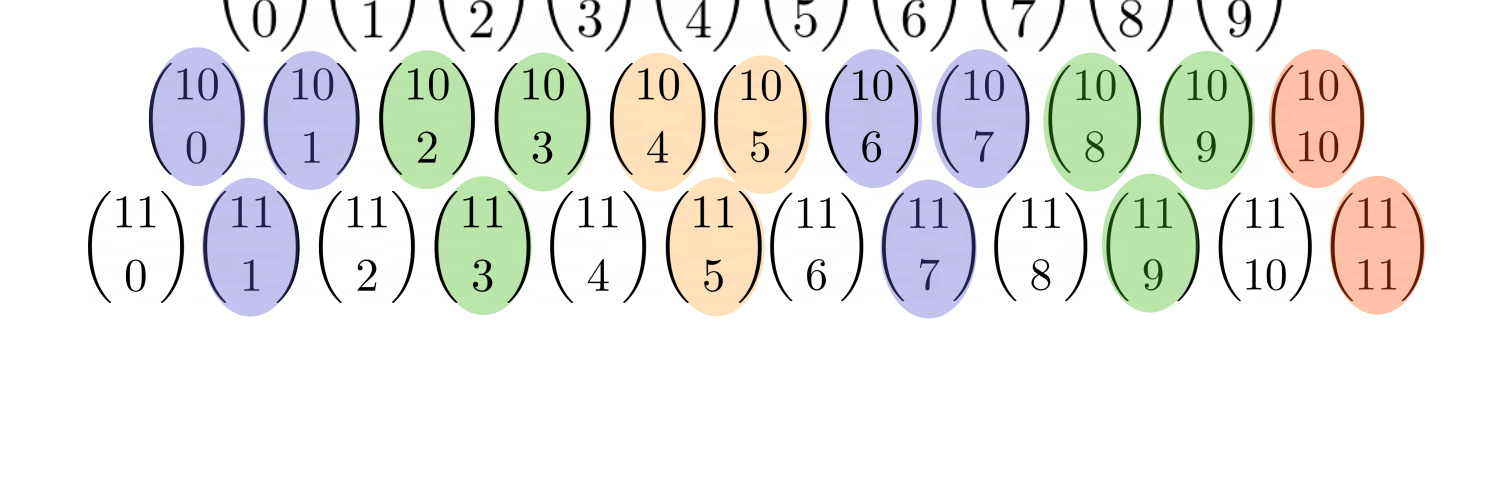# Odd and Even Sums Theorem

• Module 3 Week 2 Day 7 Challenge Part 3

The theorem says that $$\sum_{k=0}^{\lfloor{n/2}\rfloor}\binom{n}{2k}=\sum_{k=0}^{\lfloor{n/2-1}\rfloor}\dbinom{n}{2k+1}=\frac{1}{2}\sum_{k=0}^{n}\dbinom{n}{k}=2^{n-1}$$ or that the sum of chooses where the bottom is even is equal to the sum off chooses where the bottom is odd. The explanation uses binomial coefficients.

Why can't you use the fact that $$\dbinom{n}{0}=\dbinom{n-1}{0}=\dbinom{n}{n}=\dbinom{n-1}{n-1}$$ and apply $$\dbinom{n}{k}=\dbinom{n-1}{k}+\dbinom{n-1}{k-1}$$ many times to get that $$\sum_{k=0}^{\lfloor{n/2}\rfloor}\binom{n}{2k}=\sum_{k=0}^{\lfloor{n/2-1}\rfloor}\dbinom{n}{2k+1}=\sum_{k=0}^{n-1}\dbinom{n-1}{k}=2^{n-1}$$

• @spaceblastxy1428 Yes, very nice! That is a very clever and elegant way to show that the sum of the odd chooses in a row of Pascal's Triangle (or the sum of the even chooses) is equal to half of the sum of the choose in the entire row.

In this diagram below, I've highlighted the odd chooses in the 12th row:

$$\binom{11}{1}, \binom{11}{3}, \binom{11}{5}, \binom{11}{7}, \binom{11}{9}, \binom{11}{11}$$

Any choose in Pascal's Triangle is equal to the sum of the chooses directly above it, which you expressed formally as this:

$$\binom{n}{k} = \binom{n-1}{k} + \binom{n-1}{k-1}$$

Here $$(n-1)$$ refers to the row above the $$n^{\text{th}}$$ row, and the $$k+1^{\text{th}}$$ diagonal refers to the diagonal just to the left of the $$k^{\text{th}}$$ diagonal that $$\binom{n}{k}$$ is currently in.Using this formula repeatedly gives us

\begin{aligned} \textcolor{purple}{ \binom{11}{1} = \binom{10}{0} + \binom{10}{1} }\\ \textcolor{green}{ \binom{11}{3} = \binom{10}{2} + \binom{10}{3} }\\ \textcolor{orange}{ \binom{11}{5} = \binom{10}{4} + \binom{10}{5} } \\ \textcolor{purple}{ \binom{11}{7} = \binom{10}{6} + \binom{10}{7} } \\ \textcolor{green}{ \binom{11}{9} = \binom{10}{8} + \binom{10}{9} }\\ \end{aligned}

This takes care of all of the odd chooses in the $$n = 11^{\text{th}}$$ row, except for that last $$\binom{11}{11}$$ on the end. What should we do with it? We don't have two chooses on top of it, so we can't use the previous identity. And in the $$n - 1 = 10^{\text{th}}$$ row, we have a left out $$\binom{10}{10}.$$

Well, luckily we can use another identity from Pascal's Triangle! Since $$\binom{11}{11} = 1 = \binom{10}{10},$$ (the ways to take all of the things of a pile of things), then

$$\binom{10}{10} = \binom{11}{11}$$

and we are done! We've shown that every odd choose in the bottom row matches with the terms in the previous row.

Now we can use the fact that the sum of the chooses in the $$n^{\text{th}}$$ row is equal to $$2^n.$$ Thus the sum equals

$$\binom{11}{1} + \binom{11}{3} + \binom{11}{5} + \binom{11}{7} + \binom{11}{9} + \binom{11}{11} = \boxed{2^{10}}$$